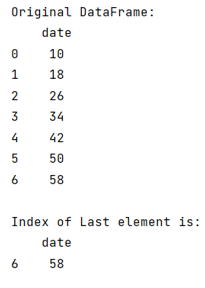# Access Index of Last Element in pandas DataFrame in Python

Given a Pandas DataFrame, we have to access index of last element. By Pranit Sharma Last updated : September 24, 2023

Pandas is a special tool that allows us to perform complex manipulations of data effectively and efficiently. Inside pandas, we mostly deal with a dataset in the form of DataFrame. DataFrames are 2-dimensional data structures in pandas. DataFrames consist of rows, columns, and data.

## Accessing Index of Last Element in pandas DataFrame

To access the last element in DataFrame, we will use pandas.DataFrame.iloc property. The iloc[] is a property that is used to select rows and columns by position/index.

Note

To work with pandas, we need to import pandas package first, below is the syntax:

```import pandas as pd
```

Let us understand with the help of an example,

## Python program to access index of last element in Pandas DataFrame

```# Importing pandas package
import pandas as pd

# Creating a dictionary
d = {"date": range(10, 64, 8)}

# Creating a DataFrame
df = pd.DataFrame(d)

# Display original DataFrame
print("Original DataFrame:\n",df,"\n")

# Access the index of last element
print("Index of Last element is:\n",df[-1:])
```

### Output

The output of the above program is: